Feasibility of singular values in the Tensor Train format

# Feasibility of singular values in the Tensor Train format

## A 4th-order example

We will go through one example of feasibility for a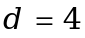th-order tensor using different algorithms. Let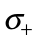be as follows:
d = 4;
sigma = cell(1,5);
sigma{1} = sqrt(13);
sigma{2} = [3,2];
sigma{3} = [sqrt(10),sqrt(2),1];
sigma{4} = [2,sqrt(3),sqrt(2.5),sqrt(2),sqrt(1.5)];
sigma{5} = sqrt(13);
sigma{:}
ans = 3.6056
ans =
3 2
ans =
3.1623 1.4142 1.0000
ans =
2.0000 1.7321 1.5811 1.4142 1.2247
ans = 3.6056

## 1. Using linear programming and hives:

For each pair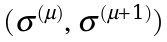we can use the linear programming algorithm linear_programming_check construct hives and determine the minimal required more size n such that the pair is feasible for that value.
help linear_programming_check
linear_programming_check investigate the feasibility of a pair (gamma,theta) linear_programming_check(n,gamma,theta,drawoptions) finds the smallest mode size m <= n for which (gamma,theta) is feasible and plots the hive obtained through the linear programming algorithm. gamma and theta must be arrays of length n but may contain zeros. Please note that this code uses honeycomb notation (not tensor notation). n is the size of the hermitian matrices. Options : drawoptions.compact == 1: less distance between honeycombs drawoptions.single == 1: also draw single honeycombs Example: gamma = sqrt([10,2,1]); theta = sqrt([4,3,2.5,2,1.5]); drawoptions = struct; drawoptions.compact = 0; drawoptions.single = 1; linear_programming_check(gamma,theta,drawoptions) --- Hive numbering and coupling (m_half = 5): each number represents a honeycomb c e | | a 2 4 gamma \ / \ / \ / upper part (gamma) 1 3 5 | | | b d f c e | | a 7 9 theta \ / \ / \ / lower part (theta) 6 8 10 | | | b d f --- Orientation of honeycombs (numbering with respect to upper part) even number: in(1) (>=0) \ / coupled(2) (>=0) | out(3) (<=0) odd number: coupled(1) (>=0) \ / in(2) (>=0) | out(3) (<=0) first: in = coupled last: out = prescribed --- Honeycomb vertex numbering, leaving out intermediate ones (n = 3): each number represents a vertex \1/ | \2/ \3/ | | \4/ \5/ \6/ | | | Y(0,0,0) (zero coordinate point is here) --- Single vertex (Y) numering: each number represents a line 1 2 \ / | 3 in direction of: 1: (0,1,-1) 2: (-1,0,1) 3: (1,-1,0) See also: test_linear_programming_check, alternating_core_generation
Be aware that indices must be shifted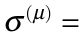sigma{mu+1}.
For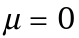and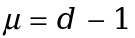however, there is nothing to do, since we know that the pair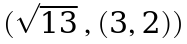is feasible for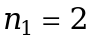and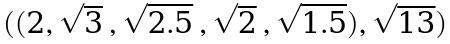is feasible forand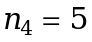(and no less) just by looking at the respective ranks.
n = [2,0,0,5];
The plots and output are nicer if the code is used outside of a live skript:
close all
drawoptions = struct;
drawoptions.compact = 0;
drawoptions.single = 0;
n(2) = linear_programming_check(sigma{2},sigma{3},drawoptions);
Warning: The problems condition is bad!
trying: 0 2 gamma = 9 4 0 theta = 1.000000e+01 2.000000e+00 1 Verification: min(A*x-b) = 8.73e-11, norm(Aeq*x-beq) = 5.59e-15 Running direct verification: iter: 0 0 1 2 3 4 5 6 7 8 9 10 11 12 13 14 15 16 17 18 19 20 21 22 23 24 25 26 27 28 29 30 31 32 33 34 35 36 37 38 39 (gamma,theta) is (numerically) feasible for n = 2 Direct verification gave error: 6.33e-15 Solution found for a minimal of 2/3 pairs of matrices: A/B_1 + A/B_2 + = diag(theta)/diag(gamma)
Boundary values (gamma, theta are squared here): a: [7 1 0] b: [3 2 0] gamma_sq: [9 4 0] theta_sq: [10 2 1]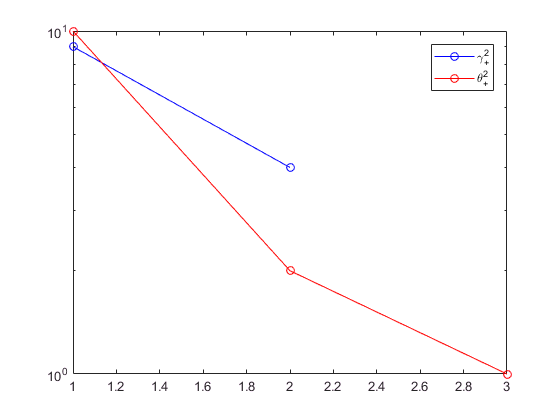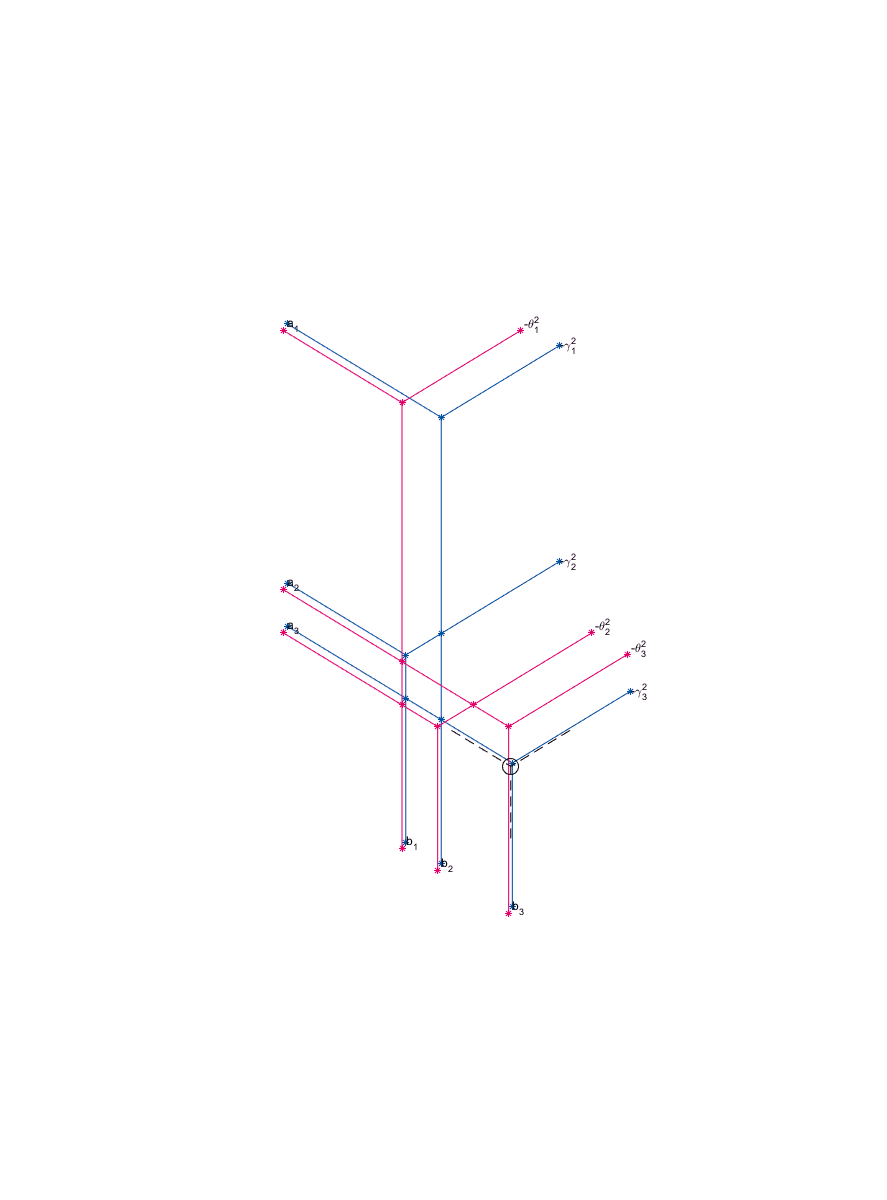drawoptions.single = 1;
n(3) = linear_programming_check(sigma{3},sigma{4},drawoptions);
Warning: The problems condition is bad!
trying: 0 2 3 4 gamma = 1.000000e+01 2.000000e+00 1 0 0 theta = 4 3.000000e+00 2.500000e+00 2.000000e+00 1.500000e+00 Verification: min(A*x-b) = 1.46e-10, norm(Aeq*x-beq) = 5.83e-15 Running direct verification: iter: 0 0 1 2 3 4 5 6 7 8 9 10 11 12 13 14 15 16 17 18 19 20 21 22 23 24 25 26 27 28 29 30 31 32 33 34 35 36 37 38 39 40 41 42 43 44 45 46 47 48 49 50 51 52 53 54 55 56 57 58 59 60 61 62 63 64 65 66 67 68 69 70 (gamma,theta) is (numerically) feasible for n = 4 Direct verification gave error: 9.44e-15 Solution found for a minimal of 4/5 pairs of matrices: A/B_1 + A/B_2 + A/B_3 + A/B_4 + = diag(theta)/diag(gamma)
Boundary values (gamma, theta are squared here): a: [2 0 0 0 0] b: [1 1 0 0 0] c: [4 0 0 0 0] d: [3 1 1 0 0] gamma_sq: [10 2 1 0 0] theta_sq: [4 3 2.5000 2 1.5000]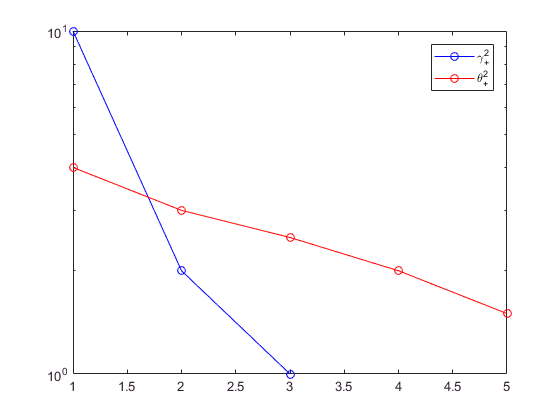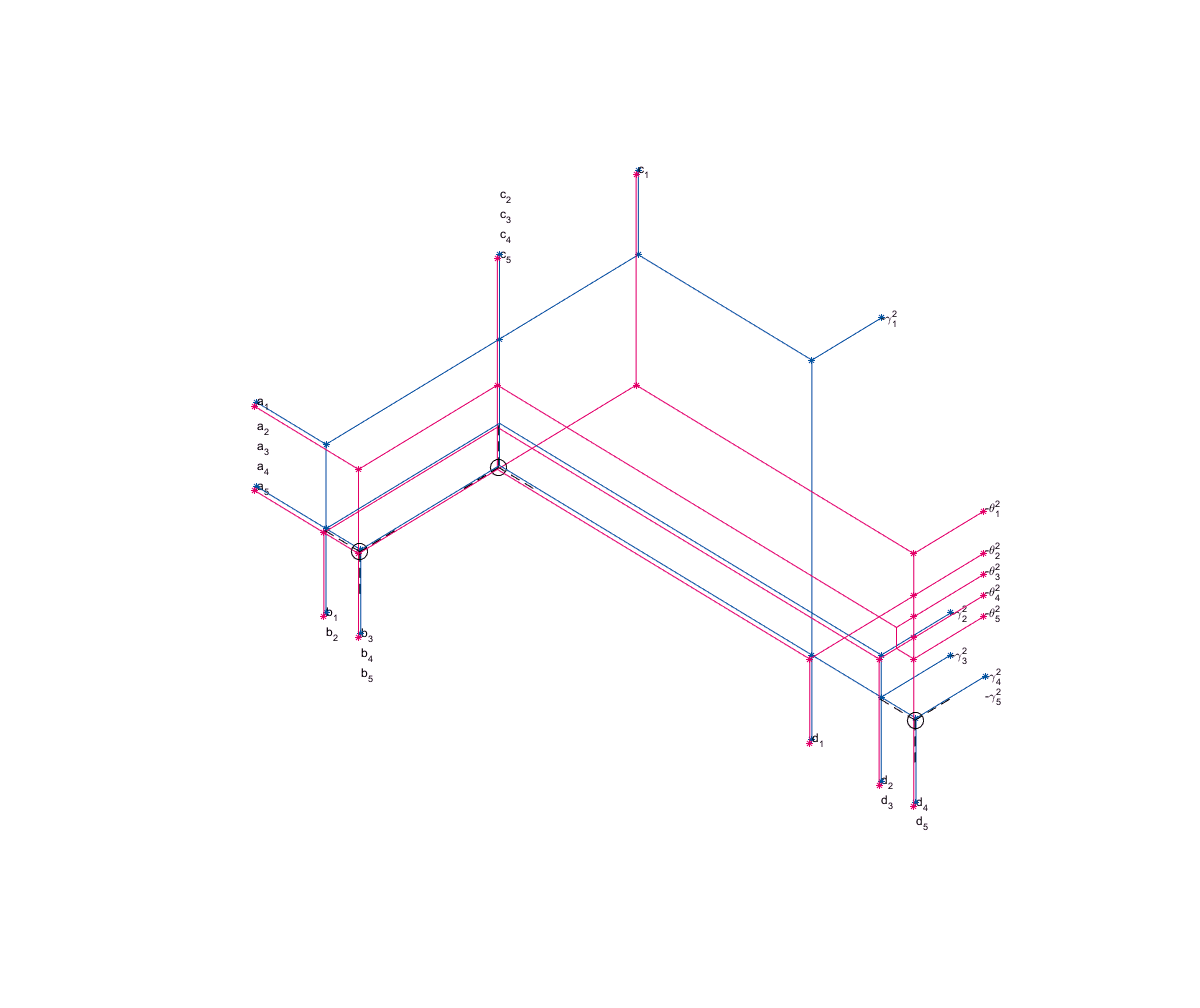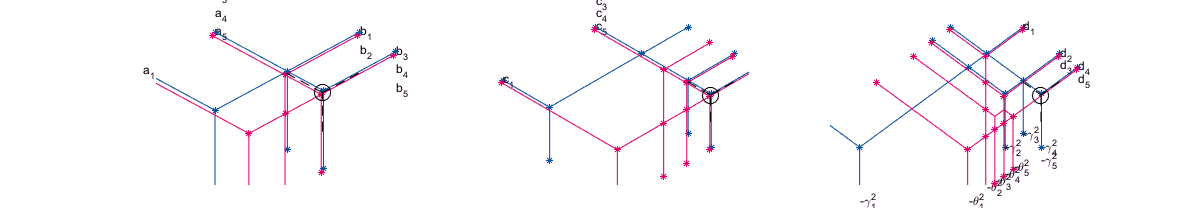Thereby we know that σ is feasible for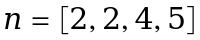and no less (except if instabilities should have corrupted our results, which is not to be expected for such small examples).
n
n =
2 2 4 5

## 2. Using the alternating iteration

For each pair we may also use the second algorithm and enforce these singular values iteratively to actually construct a representation. In order to use alternating_core_generation we need to specify a suspected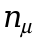.
help alternating_core_generation
alternating_core_generation checks if (gamma,theta) is feasible for n and constructs an associated core G alternating_core_generation(n,gamma,theta) takes a natural number n and a pair (gamma,theta) and checks numerically if it is feasible for n and if so, returns a core G of length n for which Gamma*G is left orthogonal and G*Theta is right-orthogonal gamma and theta do not have to be of same length and may include zeros both will be sorted in descending order alternating_core_generation(n,gamma,theta,opts) allows to specify options regarding printing and tolerance Example: gamma = sqrt([10 2 1 0 0]) theta = sqrt([4 3 2.5000 2 1.5000]) alternating_core_generation(2,gamma,theta); % fails fprintf('\n'); alternating_core_generation(3,gamma,theta); % fails fprintf('\n'); alternating_core_generation(4,gamma,theta); % succeeds fprintf('\n'); See also; test_alternating_core_generation, linear_programming_check
Again, the output is nicer if used outside of a live skript.
G = cell(1,d);
G{1} = alternating_core_generation(2,sigma{1},sigma{2});
iter: 0 0 1 (gamma,theta) is (numerically) feasible for n = 2
G{2} = alternating_core_generation(2,sigma{2},sigma{3});
iter: 0 0 1 2 3 4 5 6 7 8 9 10 11 12 13 14 15 16 17 18 19 20 21 22 23 24 25 26 27 28 29 30 31 32 33 34 35 36 37 38 39 (gamma,theta) is (numerically) feasible for n = 2
alternating_core_generation(3,sigma{3},sigma{4}); % this will fail
iter: 0 0 1 2 3 4 5 6 7 8 9 10 11 12 13 14 15 16 17 18 19 20 21 22 23 24 25 26 27 28 29 30 31 32 33 34 35 36 37 38 39 40 41 42 43 44 45 46 47 48 49 0 0 1 2 3 4 5 6 7 8 9 10 11 12 13 14 15 16 17 18 19 20 21 22 23 24 25 26 27 28 29 30 31 32 33 34 35 36 37 38 39 40 41 42 43 44 45 46 0 0 1 2 3 4 5 6 7 8 9 10 11 12 13 14 15 16 17 18 19 20 21 22 23 24 25 26 27 28 29 30 31 32 33 34 35 36 37 38 39 40 41 42 43 44 45 46 47 48 49 (gamma,theta) is likely to be not feasible for n = 3
G{3} = alternating_core_generation(4,sigma{3},sigma{4});
iter: 0 0 1 2 3 4 5 6 7 8 9 10 11 12 13 14 15 16 17 18 19 20 21 22 23 24 25 26 27 28 29 30 31 32 33 34 35 36 37 38 39 40 41 42 43 44 45 46 47 48 49 50 51 52 53 54 55 56 57 58 59 60 61 62 63 64 65 66 67 68 69 70 (gamma,theta) is (numerically) feasible for n = 4
G{4} = alternating_core_generation(5,sigma{4},sigma{5});
iter: 0 0 1 (gamma,theta) is (numerically) feasible for n = 5
The cell G now contains a representation of a tensor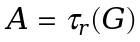which has singular values σ. Additionally,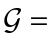G is the standard representation of A. Without previously finding n, we can also use construct_tensor_with_sv to find n and construct all cores in G in parallel.
help construct_tensor_with_sv
construct_tensor_with_sv constructs a tensor with prescribed TT-singular values in parallel construct_tensor_with_sv(n,sigma) expects a starting mode size n with n(mu) >= 1 for all mu and a cell sigma, containing TT-singular values, s.t. length(sigma) = length(n) + 1, and returns a TT-representation G with size(G{mu}) = [r(mu),r(mu)+1,n_plus(mu)] for the possible increased mode size n_plus and r(mu) = length(sigma{mu}) construct_tensor_with_sv(n,sigma,opts) specifies options for printing and tol Example (from the talk): sigma = cell(1,5); sigma{1} = sqrt(13); sigma{2} = [3,2]; sigma{3} = [sqrt(10),sqrt(2),1]; sigma{4} = [2,sqrt(3),sqrt(2.5),sqrt(2),sqrt(1.5)]; sigma{5} = sqrt(13) n = [1,1,1,1]; [G,n_plus] = construct_tensor_with_sv(n,sigma) % returns n_plus = [2,2,4,5] See also: test_construct_tensor_with_sv, alternating_core_generation
We may specify a minimal mode size to start from. We choose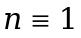here. The first call may require much more time since Matlab needs to connect to the workers in order to proceed with the parfor loop.
construct_tensor_with_sv([1,1,1,1],sigma);
Starting parallel pool (parpool) using the 'local' profile ... connected to 4 workers. sigma is (numerically) feasible for n = 2 2 4 5
In this case, the alternating iteration converges in all cases to the desired result. Note that none of the calls of alternating_core_generation need to communicate. In this sense, the algorithm runs completely parallel.

## 3. Diagonal core generation

Based on the Theorem which provides the feasibility of a pair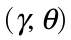for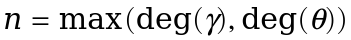, the algorithm diagonal_core_generation constructs the required core of length n.
G{1} = diagonal_core_generation(sigma{1},sigma{2});
The pair (gamma,theta) is feasible for n = 2 (or less).
G{2} = diagonal_core_generation(sigma{2},sigma{3});
The pair (gamma,theta) is feasible for n = 3 (or less).
If lucky, the required mode size turns out to be less than maximal. The algorithm will notice this (as in the following case), but still output a core of length n. It might however still not be the minimal possible mode size.
G{3} = diagonal_core_generation(sigma{3},sigma{4});
The pair (gamma,theta) is in fact feasible for ntilde = 4 (or even less).
G{3}
ans =
(:,:,1) = 0.3162 0 0 0 0 0 0.5774 0 0 0 0 0 0.0000 0 0 (:,:,2) = 0 0 0 0 0.3162 0 0 0 0 0 0 0.5774 0 0 0 (:,:,3) = 0 0 0 0.3162 0 0 0 0 0 0 0 0 0 0 0 (:,:,4) = 0 0 0.3162 0 0 0 0 0 0 0 0 0 0 0 0 (:,:,5) = 0 0 0 0 0 0 0 0 0 0 0 0 0 0 0
G{4} = diagonal_core_generation(sigma{4},sigma{5});
The pair (gamma,theta) is feasible for n = 5 (or less).
Again, the cell G now contains the standard representation of the tensorwhich has singular values σ. This time, all cores are rather sparsely populated.